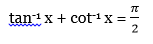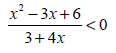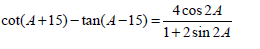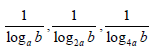# UP Board Important Questions for Class 11 Maths

The UP Board Class 11 annual exams are near to its conduct. Mostly the UPMSP conduct the Class 11 UP Board Exams in the month of March. Students who are aiming to score good marks in the exams should start solving the important questions, at least 15 days prior to the annual exam. And in the case of Mathematics subjects, it should be even before that. So, to make your preparation in a better way, here we are providing the UP Board Class 11 Maths Important Questions. These Questions cover the all the important topics of UP Board Class 11 Maths Syllabus.

We all know that Maths is all about practising. The more you practice, the more your concept will build up. Moreover, you would be able to solve the problem in the easiest way. So, the best way to test your UP Board Class 11 Maths preparation is to practice the important questions from the UP Board Class 11 Previous Year Questions Papers or sample papers. Further, on the page, you will find all the important UP Board Class 11 Maths Questions.

### How to practice UP Board Class 11 Maths Important Questions

Here are a few points that you should follow before solving these important UP Board Maths Questions.

1. Do not look for the solutions, while practising these questions.
2. Be honest to yourself. Allocate a time duration to yourself before starting to solve.
3. Once you are done with the questions, evaluate yourself.
4. Go through the solutions of those questions which you would be able to solve.

Here we have provided the set of UP Board Class 11 Maths Important Questions that consist of long answers.

The Questions number from 1 to 10 below carries 4 marks each:

Q1. Solve sin2 x + sin2 2x = 1

Q2. Find the value of i30 + i40 + i60

Q3. Prove by mathematical induction that 11n+2 +122n+1 is divisible by 133 for all positive integer values of n.

Q4. Determine whether the points (0,0) and (5,5) lie on different sides of the straight line x + y -8 = 0 or on the same side of the straight line.

Q5. Prove thatQ6. A, B, C are 3 sets and U is the universal set such that

n(U) = 800, n (A) = 200, n(B) = 300 ,n(AB) =100 Find n(A’B’)

Q7. If P be the sum of the odd terms and Q the sum of the even terms in the expansion of (x + a)n , prove that P2 – Q2 = (x2 – a2 )n

Q8. If α, β are the roots of the equation x2 – bx + c = 0 find the value of α2 + β2

Q9. Solve the inequalityQ10. Prove that:The Questions number from 11 to 17 below carries 7 marks each:

Q11. The mean and variance of 7 observations are 8 and 19 respectively. If 5 of the observations are 2, 4, 12, 14, 11. Find the remaining observations.

Q12. How many 6 digits numbers can be formed with the digits 1, 2, 3, 4, 5, 6, 7 if the 10th, unit’s places are always even and repetition is not allowed?

Q13. In the expansion (1+ x)40, the coefficients of T2r+1 and T r+2 are equal, find r?

Q14. Differentiate log10 x with respect to x.

Q15. On the average one person dies out of every 10 accidents find the probability that at least 4 will be safe out of 5 accidents.

Q16. Shift the origin to a suitable point so that the equation x2 + y2 – 4x + 6y = 36 representing a circle is transformed into an equation of a circle with centre at the origin in the new coordinate axes.

Q17. Prove thatform an AP.